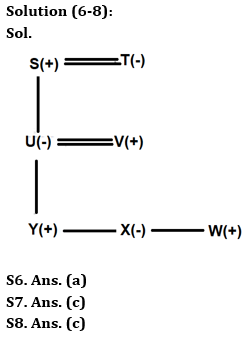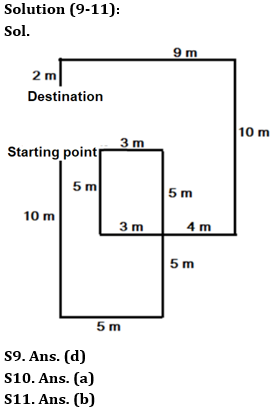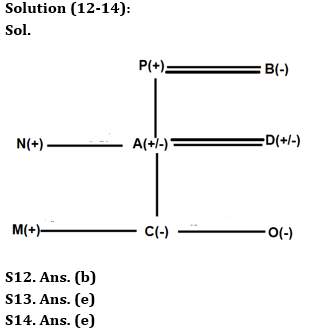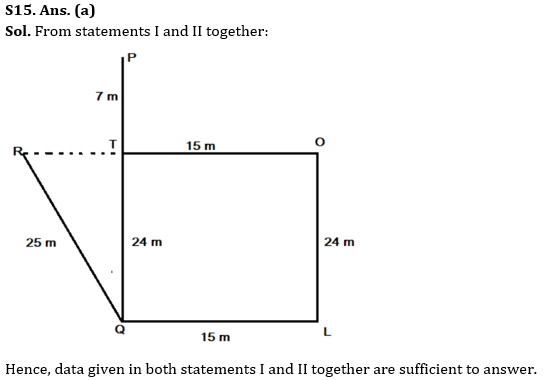Latest Banking jobs   »

# Reasoning Quiz For RBI Grade B Phase 1 2023-31st March

Directions (1-5): The questions below consist of three statements numbered I and II given below it. You have to decide whether the data provided in the statements are sufficient to answer the question. Read all the statements and answer the question.

Q1. There are 9 persons P, Q, R, S, T, U, V, W, and X sit in row facing north direction. How many persons sit between R and S?
Statements:
I. Three persons are sitting between U and W, who is sitting 2nd to the left of X. Q is sitting 3rd to the left of R, who is sitting to the right of U. Q is not sitting adjacent to U.
II. One person is sitting between W and T. One person is sitting between P and T. S is not sitting adjacent to X.
(a) Data given in both statements I and II together are sufficient to answer.
(b) Data given in statement I alone is sufficient to answer.
(c) Data given in statement II alone is sufficient to answer.
(d) Data given in both statements I and II together are not sufficient to answer.
(e) None of the above

Q2. Six persons A, B, C, D, E, and F are standing in a row facing north in increasing order of their heights from right to left. Who among the following is second tallest?
Statements:
I. C’s height is 150cm. A is not immediate neighbour of E. F is not shortest.
II. More than two persons are standing to right of A. B and D are taller than C.
(a) Data given in both statements I and II together are sufficient to answer.
(b) Data given in statement I alone is sufficient to answer.
(c) Data given in statement II alone is sufficient to answer.
(d) Data given in both statements I and II together are not sufficient to answer.
(e) None of the above

Q3. V, W, X, Y, and Z are five friends who live on different floor of a 9-floor building such that the ground floor is 1, just above it is numbered as 2 and so on till the topmost floor is 9. Some floors are vacant. Y lives on which floor, if W doesn’t live on 3rd & 5th floor?
Statements:
I. Z lives on odd numbered floor. Y neither lives immediately above Z nor immediately below V. W lives below X. V lives on odd number floor.
II. X lives on even number floor below V. W does not live on 1st or 4th floor. Two persons live between V and X.
(a) Data given in both statements I and II together are sufficient to answer.
(b) Data given in statement I alone is sufficient to answer.
(c) Data given in statement II alone is sufficient to answer.
(d) Data given in both statements I and II together are not sufficient to answer.
(e) None of the above

Q4. In a family of 9 members i.e., G, H, I, J, K, L, M, N, and O has three generations. How is N related to J?
Statements:
I. I is the unmarried brother of G. L is the sister-in-law of G. There are two married couples in the family.
II. N is not married to L. O has only one daughter. M is not a female. H is the child of N.
(a) Data given in both statements I and II together are sufficient to answer.
(b) Data given in statement I alone is sufficient to answer.
(c) Data given in statement II alone is sufficient to answer.
(d) Data given in both statements I and II together are not sufficient to answer.
(e) None of the above

Q5. Six persons E, F, G, H, I and J have different heights. How many persons are taller than H?
Statements:
I. F is taller than E and J. Neither G nor H is shorter than F. I is shorter than E but not shortest. H is not the tallest person.
II. At least three persons are shorter than H. E is shorter than G. F is not shorter than I.
(a) Data given in both statements I and II together are sufficient to answer.
(b) Data given in statement I alone is sufficient to answer.
(c) Data given in statement II alone is sufficient to answer.
(d) Data given in both statements I and II together are not sufficient to answer.
(e) None of the above

Direction (6-8): Answer the questions based on the information given below.
There are 7 members in a family which consists of 3 generations. The family consists of 2 married couples. X is the only daughter of V, who is the son in law of T. U is the only daughter of S, who is the grandfather of W. U has 3 children. Y is one of the members in the family.

Q6. How is Y related to S?
(a) Grandson
(b) Son
(c) Grand daughter
(d) Nephew
(e) Can’t be determined

Q7. How is the wife of father-in-law of V related to Y?
(a) Mother
(b) Sister
(c) Grandmother
(d) Paternal Aunt
(e) Can’t be determined

Q8. How many female members are there in the family?
(a) One
(b) Two
(c) Three
(d) Four
(e) None of these

Direction (9-11): Answer the questions based on the information given below.
Aarush walks 10m from his home in south direction. He then takes a left turn and walks for 5m. He then walks 10m in north direction and then takes a left turn and walks 3m. He then turns south and walks 5m and then again takes a left turn and walks 7m. He then walks 10m and 9m in north and west direction respectively. Finally, he takes a left turn and walks 2m to reach his destination.

Q9. What is the direction of Aarush’s destination with respect to his starting point?
(a) South
(b) North-east
(c) South-west
(d) North
(e) East

Q10. What is the shortest distance between his last point and starting point?
(a) 3m
(b) 5m
(c) 6m
(d) 8m
(e) None of the above

Q11. Aarush takes how many turns from in his total travelling from his house to his destination?
(a) 9
(b) 8
(c) 10
(d) 7
(e) None of the above

Directions (12-14): Answer the following question based on the information given below:
A, B, C, D, M, N, O and P are eight members in a family. There is no single parent in the family. P is the father of A. N is the uncle of C. A and D are married couple. M is the only son of D. P has only two children. C is the granddaughter of P. O is not married to P or N. D has no siblings. C has one sister.

Q12. How is C related to B?
(a) Daughter
(b) Grand-daughter
(c) Grandmother
(d) Sister
(e) None of these

Q13. How is A related to O?
(a) Father
(b) Daughter
(c) Mother
(d) Son
(e) Either ‘Father’ or ‘Mother’

Q14. How many male members are there in the family?
(a) One
(b) Two
(c) Three
(d) Four
(e) Can’t be determined

Q15. The questions below consist of two statements numbered as I and II. You have to decide whether the data provided in the statements are sufficient to answer the question. Read all the statements and answer the question.
Six people L, O, P, Q, R, and T are sitting at some distance from each other. What is the distance of T with respect to R?
Statements:
I. Q is sitting 25 m to the southeast of R. O is sitting 15 m to the east of T. R is sitting to the west of T. P is sitting 31m to the north of Q.
II. T is sitting at the point of intersection of line joining RO and PQ. L is sitting to the south of O and to the east of Q. Distance between O and L is 24 m.
(a) Data given in both statements I and II together are sufficient to answer.
(b) Data given in statement I alone is sufficient to answer.
(c) Data given in statement II alone is sufficient to answer.
(d) Data given in both statements I and II together are not sufficient to answer.
(e) None of the above

Solutions

Solution (1-5):
S1. Ans. (d)
Sol. From both statements, we can’t get the answer. So, data given in both statements I and II together are not sufficient to answer.

S2. Ans. (d)
Sol. From both statements, we can’t find the answer. So, data given in both statements I and II together are not sufficient to answer.

S3. Ans. (d)
Sol. From both statements, we can’t get the answer. So, data given in both statements I and II together are not sufficient to answer.

S4. Ans. (d)
Sol. From both statements, we can’t find out the relation between N and J. Hence, data given in both statements I and II together are not sufficient to answer.

S5. Ans. (b)
Sol. From Statement I, F is taller than E & J. Neither G nor H is shorter than F. I is shorter than E but not shortest. H is not the tallest person, so, G > H > F > E > I > J
So, only one person is taller than H. Hence, data given in statement I alone is sufficient to answer.## FAQs

### What is the selection process of the Bank Clerk?

There are 4 sections in the RBI Grade B Phase 1 Exam i.e. English Language, General Awareness, Quantitative Aptitude & Reasoning.

#### Congratulations!Union Budget 2023-24: Free PDF